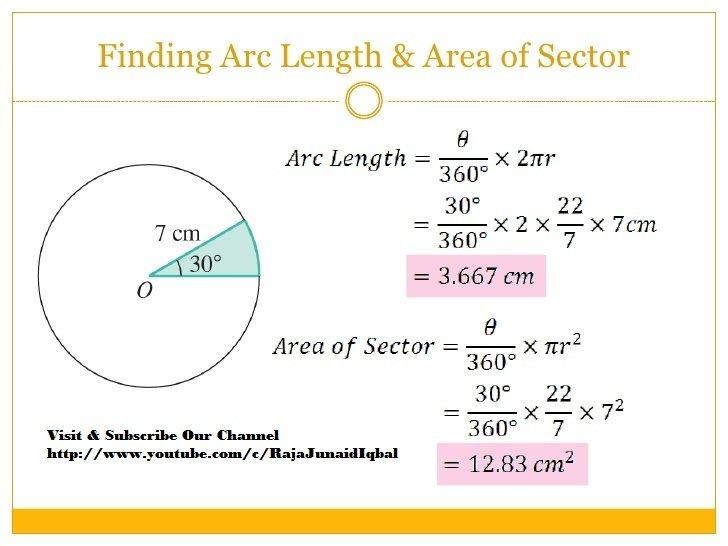Breaking News
Home / Land Surveying / Finding Arc Length and Area of Sector

# Finding Arc Length and Area of Sector

## Finding Arc Length and Area of Sector

What is the formula for the area of a sector of a circle?
If you’re asking for the area of the sector, it’s the central angle of 360, times the area of the circle, for example, if the central angle is 60, and the two radiuses forming it are 20 inches, you would divide 60 by 360 to get 1/6

### Thumb Rules For Civil Engineers In Building Construction

What is the formula for the arc length of a circle?
To find arc length, start by dividing the arc’s central angle in degrees by 360. Then, multiply that number by the radius of the circle. Finally, multiply that number by 2 × pi to find the arc length. If you want to learn how to calculate the arc length in radians, keep reading the article.### THANKS.